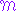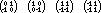## CIS-3353  Programming Languages & Artificial Intelligence

SBU policy is that they own the Syllabus; other material is the property of the instructor who prepared it.

Assignments

Feb.10: p.149, #4,5,7,10,14,19, (12e,12f,15d,15e, but for Java not C)

Feb.17: p.177, #1,3,5; p.213, #4,12

Mar.3: p.277, #2,5,14,15,22

Mar.10: Write a critique of Turk/2, using all relevant evaluation criteria. Automatic A for the first half of the semester if your paper is publication quality (in the instructor's opinion, or else gets accepted for publication in a qualified journal). You may encorporate some or all of the posted White Paper for a co-authored publication paper. You may turn in draft copies of this paper as many times as you like; the final draft on March 10 is graded.

Mar.24:  Write a program to implement the Sieve of Eratosthenes algorithm for finding and printing out all the prime numbers  <100. Turn in source code and output for each version:

a. In Java
b. In Visual Basic (or see instructor for alternative language)
c. Smalltalk (due Mar.26)
Apr.7:   d.  The same Sieve of Eratosthenes algorithm in Scheme.

Apr.23:  e.  The same Sieve of Eratosthenes algorithm in Prolog.

Apr.30:  AIT&P exercises, p.122 #3.2; p.171 #4.1. In preparation for #4.1, you might experiment with the files "grids.scm" and "search.scm" (both on the G:\ drive in folder "G:\CLASS\Computer Science\CIS3353\SchemeLisp") to see how to write Scheme functions for some of the other search algorithms in this chapter (note that not all of the sample code works properly in DrScheme; for example, the "best" algorithm as coded fails because we have no sort2 function). After you have implemented your hill-climbing-search algorithm and shown it to fail on the included culdesac data grid, construct a grid representing something like Fig.4.12 (p.147) and show that your algorithm solves it correctly.

May 12: Do AIT&P exercises on p.243 #5.1, 5.4, 5.8, 5.10, 5.11, and 5.14; turn in your completed solutions to 5.1 and 5.11. Show that you understand what is involved in doing the remaining four, because you need to understand these ideas for this course (that is, for the final) and now is a good time to get them under control. I have prepared some Scheme utility functions in "IsoRect.scm" to help with #5.1, and have adjusted the author-supplied files "Version.scm" (for #5.4), "Decision.scm" (for #5.8), "pdp.scm" (for #5.10, but load "pdp1.scm" or "pdp2.scm" to use it), "Perceptron.scm" (for #5.11), and "Genetic.scm" (for #5.14, from chapter 4), all now on the G:\ drive in folder "G:\CLASS\Computer Science\CIS3353\SchemeLearning", to make it easier to use them.

May 14: AIT&P exercises, p.289, #6.3, 6.6 (see file "TM.scm" on the G:\ drive in folder "G:\CLASS\Computer Science\CIS3353\SchemeLisp"), 6.9, 6.12, and p.349 #7.7, 7.10, 7.15.

#### Notes on May 14 assignment:

6.3.  You need to be careful with your categories. A predicate like "holds" is true (or false) for all time, given its particular parameters. A situation "s" is like a point in time, at which time a predicate like "holds" can meaningfully be true (or false) for some fluent. Fluents are like predicates or variables (except that they can go on or off at points in time, while predicates are always true or false for all time). Events like "toggle" are not fluents nor predicates; they cannot be true or false. However, a result of an event in a particular situation is another situation. In this problem, 'abnormal' is a fluent: maybe it is true, maybe it's not, depending on the situation. The on state of the light and the on state of the switch are also fluents. Power failure and restoration are events. Accordingly, for all situations s, each of the following implications is true:
holds(s,on(switch) ^ normal) => holds(s,on(light)) -- if the switch is on and power is normal, the light is on
holds(s,on(switch)) => holds(result(s,toggle), not(on(switch))) -- toggle turns on to off or off to on...
holds(s,not(on(switch))) => holds(result(s,toggle), on(switch))
holds(s,not(normal)) => holds(s,not(on(light))) -- abnormal power implies the light is off, regardless the switch
holds(result(s,restore),normal) -- the result of restoring the power is that it is once again normal

6.6.  Contra has already been altered in TM.scm, all you have to do is specify a causal rule that uses it. I did it this way:

(pcause (location R x) (move R x y) (location R y))
which can be read as "if R is at location x, then event 'move R from x to y' has the effect of making the location of R to be y." This can be easily tested with the following code:
(let ((initial-conditions '((location R x)))
(initial-events '(((move R x y) 9)))
(theory '((pcause (location R x) (move R x y) (location R y)) )) )
(tm initial-conditions initial-events theory)
(display-tm))

6.9.  Let a be Fred (an agent), and let q be "his neighbor's house is on fire", and f be "the right thing to do is call the fire department." The proof follows immediately from the first axiom on p.275.

7.7.  LCP is of no help at all, since you cannot re-order your travel from point A to point B and from thence to point C. A schema:

(make-operator '((location (? a) (? x)) (nextto (? x) (? y)))
'((location (? a) (? y))) ;; addition
'((location (? a) (? x))))  ;; deletion

7.10. I think I would bring in the situation calculus from ch.6, and define events to be prevented (like the termination of a condition to be maintained). Prevention occurs when a condition (fluent) holds at a situation where where otherwise the preventable event would occur. Such fluents normally are the result of other events -- such as studying for an exam to prevent failing it -- but the studying can happen at any time during the week before the time of the exam, so LCP is definitely valuable here.

7.15.  Repeat-until-done actions can be encoded with a conditional plan based on the done-ness, which is satisfied or enables further steps (swinging the hammer back for another blow) leading to satisfaction.

#### Final Exam, with Answers (in color)

1. What is a by-name parameter, and how is it different from the parameter passing protocols common in modern languages like C and Java? Describe or show how you might get the same effect and functionality in pure Java of the name parameters in the following code:
int n, maxa;
float a[];

class int_BYNAME {int get(){return 0;}}     // superclass
class flt_BYNAME {float get(){return 0.0;}} // superclass
class n_star_2 extends int_BYNAME {int get(){return n*2;}}
class n_div_2 extends int_BYNAME {int get(){return n/2;}}
class a_maxa_n extends flt_BYNAME {float get(){return a[maxa-n];}}
class a_n extends flt_BYNAME {float get(){return a[n];}}

void tricky(int_BYNAME k, float_BYNAME x)
{ for (int i=0; k.get()<maxa; ++i) {n+=i; a[k.get()]=x.get()*2.0;} }
Ö
n=0;
tricky(n*2,a[maxa-n]); tricky(new n_star_2(),new a_maxa_n());
tricky(n/2,a[n]);    tricky(new n_div_2(), new a_n());
Ö

Most modern languages like C and Java pass all parameters by value, which means a copy of the value is passed to the function. C can explicitly pass pointers to parameter values, which gives the effect of passing by reference. Java does not have general pointers, so you can only get that effect with object wrappers.

By-Name parameters are evaluated only at the time they are actually used, which means that the call protocol must include a thunk (anonymous function) to do the evaluation. We get this effect by subclassing a wrapper class and overriding its get() method with the specifics of this thunk.

This problem derived from item #10 on the study guide, on the semantics of parameters that may be passed.

2. Describe or show a simple way to solve the same by-name problem in Scheme:

The point of this problem is to demonstrate familiarity with l-calculus (study guide item #13)

(define n 0)
(define maxa (something))
(define a (list . . .))
(define (tricky k-by-name x-by-name)

(let ((value-of k (___________________________________________________))

(do ((i 0 (+ i 1)))
((>= (value-of k) maxa))
(set! n (+ n i))
(set! (nth (k) a) (* (x) 2.0))))
Ö

(tricky (lambda () (* n 2)) (lambda () (nth (- maxa n) a)))

(tricky (lambda () (/ n 2)) (lambda () (nth n a)))
Ö
)

3. Explain the difference between axiomatic, denotational, and operational semantics by showing how each would apply to the following line:
int A = 3;
Operational semantics computes the value 3 and stores it into the variable A.

Axiomatic semantics offers a minimal precondition of {} (empty set), and a postcondition {A=3}.

Denotational semantics starts with an initial environment where A is undefined (or perhaps not yet even in the set), and defines the Meaning functionas a new environment thus:(int A=3, {(A,?)}) = {(A,3)}
This problem appeared on the first midterm; it is derived from study guide item #1.

4. You have been assigned responsibility for the graphics transmission part of a teleconferencing software package that supports line drawings and works over low-speed modems. It has been determined that sending bitmaps of the scanned line drawings is too slow, but it is believed the drawings can be decomposed into one or more individual image components, each of which can be approximately described parametrically by the following equation:

ax2 + by2 + cxy + dx + ey + f = 0
where the coefficients a, b, c, d, e, and f  are float values (possibly 0). From what you learned in this course discuss how you might go about finding these coefficients from the scanned image.

This problem is derived from study guide item #17 on computer vision and AI solutions to its peculiar problems, and from study guide item #15 on search strategy. You should be thinking in terms of edge detection analysis by applying matrices. Then you should be thinking in terms of a search heuristic for finding the six parametric coefficients describing each image component. I would use a hill-climbing heuristic with an altitude function that measures closeness of fit, perhaps least-squares distance from the formula to the nearest edge in the image. A good multiple-parameter hill-climbing heuristic is a so-called genetic program, where the coefficients represent the "alleles" and the least-squares hill maxima the "fitness". Some appropriate randomizing function will be needed to tweak the coefficients in search of local maxima, with "anealing" to effect leaps to other non-local maxima.

5. The following is an abbreviated BNF grammar for an obscure (untyped) string-based programming language. Show your understanding of language principles by writing a function in this language to compute n! and enough of an additional subprogram to build a table of the first 8 factorials by calling your function. Recall that factorial n (spelled ìn!î) is the product of all the integers from 1 to n:

 ::=  "function" ()* "end" ::=  ("," )* ::=  ::=  "put" "into" ::=  "if" "then"   ()* "else" ()* "end" "if" ::=  "if" "then"   ()* "end" "if" ::= "repeat" "with" "=" "to" ()* "end" "repeat" ::= "return" ::= | | ::= ::= "(" ")" ::= "(" ::= ("," )* ")" ::= ")" ::= ::= "of" ::= "line" | "word" ::= "+" | "?" | "*" | "/" | "is" | "<" | ">" | "*"

This problem is intended to demonstrate the ability to read and understand grammars based on BNF (study guide item #1), which is a valuable skill a good programmer needs from time to time in the workplace. It turned out to be surprisingly difficult for students to refrain from adding to their program syntactic elements forbidden by the grammar, such as extra parenthesis. Although it was not necessary, extra points were awarded for a recursive solution:

function factorial n
if n>1 then
return factorial(n-1)*n
else
return 1
end if
end factorial

...
put "" into table
repeat with ix=1 to 8
put ix into word 1 of line ix of table
put factorial(ix) into word 2 of line ix of table
end repeat

Rev.  2003 May 23